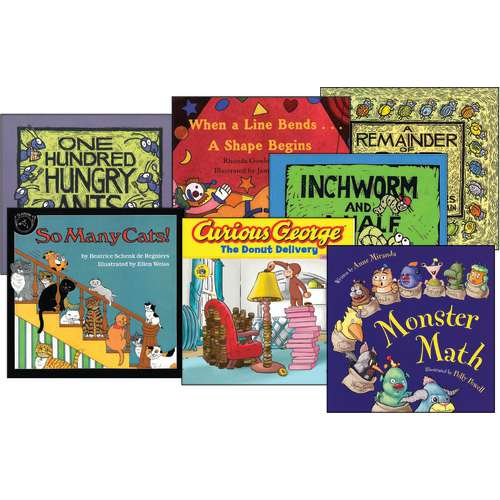•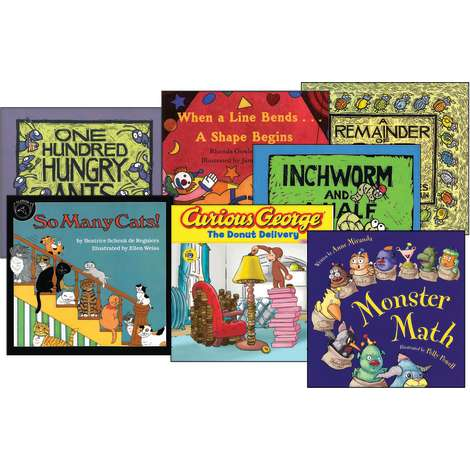•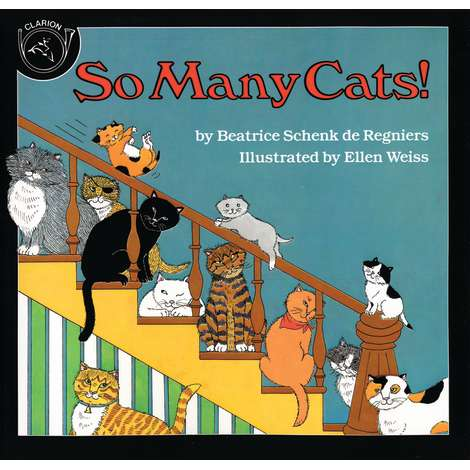•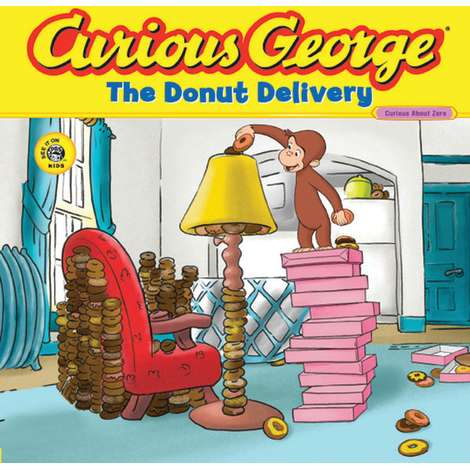•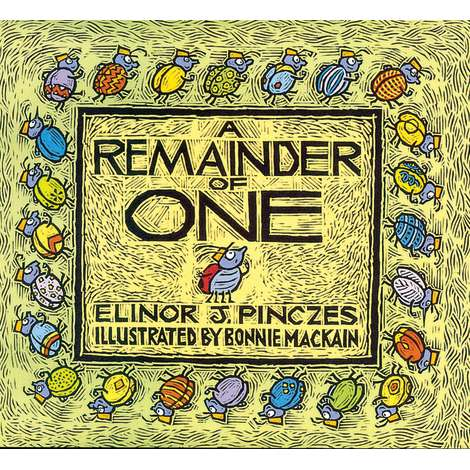•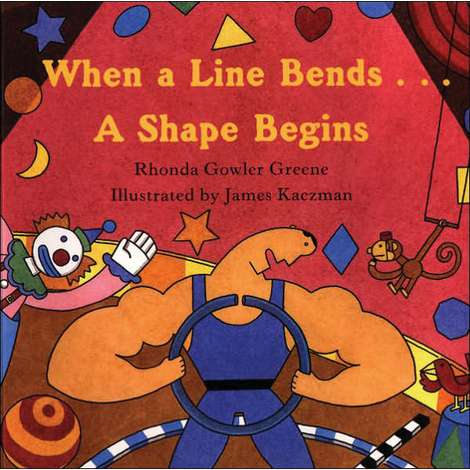•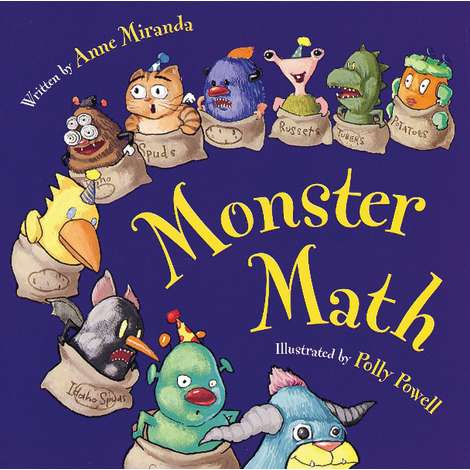•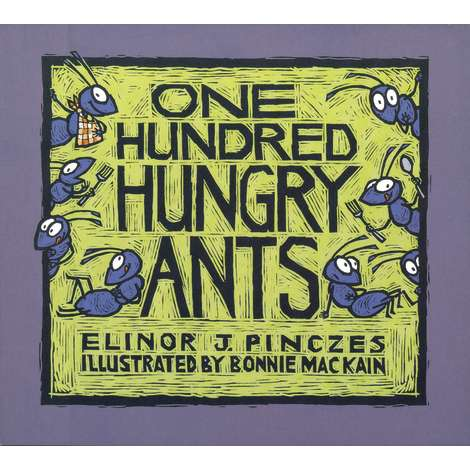•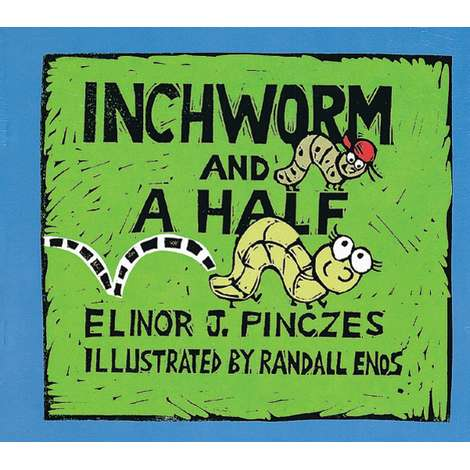Math Literature Set, Set of 7 books

Product Number: HOU9780618891122
\$45.82
7 easy to read math stories in one convenient collection that introduce mathematical concepts in a format young learners can ...
Quantity

7 easy to read math stories in one convenient collection that introduce mathematical concepts in a format young learners can comprehend. Curious George's Donut Delivery, Inchworm and a Half (measurement), Monster Math, One Hundred Hungry Ants (division), So Many Cats (counting), Remainder of One (division, problem solving), and My Full Moon is Square (square roots).
HOU9780618891122